# US5956702A - Time-series trend estimating system and method using column-structured recurrent neural network - Google Patents

Time-series trend estimating system and method using column-structured recurrent neural network Download PDF

## Info

Publication number
US5956702A
US5956702A US08/701,700 US70170096A US5956702A US 5956702 A US5956702 A US 5956702A US 70170096 A US70170096 A US 70170096A US 5956702 A US5956702 A US 5956702A
Authority
US
United States
Prior art keywords
time
value
data
series
estimating
Prior art date
Legal status (The legal status is an assumption and is not a legal conclusion. Google has not performed a legal analysis and makes no representation as to the accuracy of the status listed.)
Expired - Fee Related
Application number
US08/701,700
Inventor
Masahiro Matsuoka
Mostefa Golea
Current Assignee (The listed assignees may be inaccurate. Google has not performed a legal analysis and makes no representation or warranty as to the accuracy of the list.)
Fujitsu Ltd
Original Assignee
Fujitsu Ltd
Priority date (The priority date is an assumption and is not a legal conclusion. Google has not performed a legal analysis and makes no representation as to the accuracy of the date listed.)
Filing date
Publication date
Priority to JP7-229509 priority Critical
Priority to JP7229509A priority patent/JPH0973440A/en
Application filed by Fujitsu Ltd filed Critical Fujitsu Ltd
Assigned to FUJITSU LIMITED reassignment FUJITSU LIMITED ASSIGNMENT OF ASSIGNORS INTEREST (SEE DOCUMENT FOR DETAILS). Assignors: MATSUOKA, MASAHIRO, GOLEA, MOSTEFA
Application granted granted Critical
Publication of US5956702A publication Critical patent/US5956702A/en
Anticipated expiration legal-status Critical
Application status is Expired - Fee Related legal-status Critical

## Images

•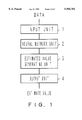•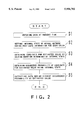•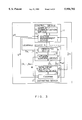•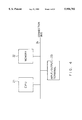•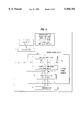•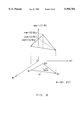•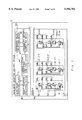•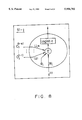•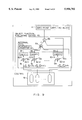•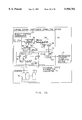•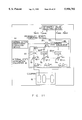•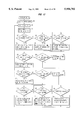•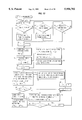•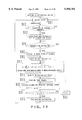•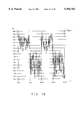•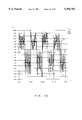•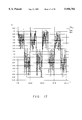•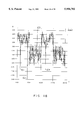••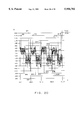•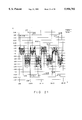•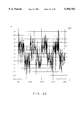•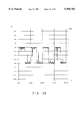•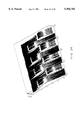•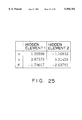•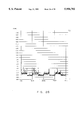•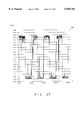•••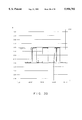••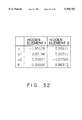•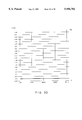•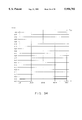•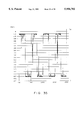•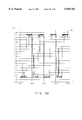•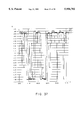•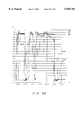•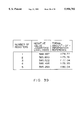## Classifications

• GPHYSICS
• G06COMPUTING; CALCULATING; COUNTING
• G06NCOMPUTER SYSTEMS BASED ON SPECIFIC COMPUTATIONAL MODELS
• G06N3/00Computer systems based on biological models
• G06N3/02Computer systems based on biological models using neural network models
• G06N3/04Architectures, e.g. interconnection topology
• G06N3/049Temporal neural nets, e.g. delay elements, oscillating neurons, pulsed inputs

## Abstract

Each neural element of a column-structured recurrent neural network generates an output from input data and recurrent data provided from a context layer of a corresponding column. One or more candidates for an estimated value is obtained, and an occurrence probability is computed using an internal state by solving an estimation equation determined by the internal state output from the neural network. A candidate having the highest occurrence probability is an estimated value for unknown data. Thus, the internal state of the recurrent neural network is explicitly associated with the estimated value for data, and a data change can be efficiently estimated.

## Description

BACKGROUND OF THE INVENTION

1. Field of the Invention

The present invention relates to a recurrent neural network, and also to a system and method of estimating the trend of a change in measurement discontinuously variable in time.

2. Description of the Related Art

The Kalman filter has been conventionally used as an estimation filter. It is a classic method of identifying a system, and is still being developed for various applications. There are various methods of applying a neural network being newly established as a static nonlinear system identifying method for estimating nonlinear time-series data. However, the application of the Kalman filter is limited by the capabilities of the current computers, and the applications relating to the time-series analysis of the neural networks are new and have the following problems.

(1) Method using Kalman Filter

When discontinuous changes are made in the trend of time-series data or when a Gaussian type cannot be estimated for the noise representing the uncertainty of a model, a normal linear Gaussian Kalman filter does not properly estimate or filter data (refer to Time-series Analysis Programming by Genshiro Kitagawa, in Iwanami Computer Science published in 1993). If a discontinuous state change is processed by a linear Gaussian model, then an extremely large dimensional model is required. At this time, it is difficult to set a standard for objectively selecting the dimension of the model.

Recently, to solve the above described problems, a nonlinear and non-Gaussian generalized Kalman filter has been introduced (Genshiro Kitagawa. Non-Gaussian State-Space Modeling of Nonstationary Time Series. Journal of the American Statistical Association, 82 (400): 1032-1041, 1987.). The generalized Kalman filter is successfully used in estimating and smoothing a discontinuous change in trend or a noise. However, to operate the generalized Kalman filter, the distribution of the estimating, filtering, and smoothing should be directly computed. Therefore, when a larger state-space model is used, it takes a longer time in identifying a filtering coefficient appropriate for the generalized Kalman filter. On the other hand, since the probability distribution required for the computation in each step can be determined using the linear Gaussian model only by estimating a mean value and distribution, the volume of computation for the identification can be reduced but identification objects are limited. Additionally, to effectively apply the generalized Kalman filter, prior knowledge is required for the distribution in which noises including an abnormal value can be appropriately represented.

However, using a Monte Carlo filter for estimating the distribution of the noises from a sample in the bootstrap method, data can be appropriately estimated or smoothed even if prior knowledge is lacking for noises (Genshiro Kitagawa. A Monte Carlo Filtering and Smoothing Method for Non-Gaussian the receives Nonlinear State Space Models. Research Memorandum 462, The Institute of Statistical Mathematics, 12 1993.). Using the Monte Carlo filtering method, a methodology for common nonlinear non-Gaussian time series data is being established. However, the time required in computing the probability distribution through a resampling process is significantly lengthened.

(2) Method using a Neural Network

Data is retrieved from time-series data through a time window. Then, a series of patterns are generated to indicate small differences in a time series so that the time series data are learned through the feed-forward neural network and back propagation (A. Waibel. Modular Construction of Time-Delay Networks for Speech Recognition. Neural Computation, 1:382-399, 1989./Jeng-Neng Hwang, Shyh-Rong Lay, Martin Maechler, R. Douglas Martin, and James Schimert. Regression Modeling in Back-Propagation and Projection Pursuit Learning. IEEE Transactions on Neural Networks, 5(3):342-353, May 1994.). There is the problem in exactly learning the time series data in this method that the scale of the neural network is large and the storage areas are running short. The problem is caused by representing the relationship between input and output data by a weight value of the neural network. Furthermore, another problem arises. That is, definite descriptions cannot be made from the viewpoint of generating a probability structure of time-series data.

To solve the problems of network scales, a recurrent neural network having a feedback structure has been designed (Jerome T. Connor, R. Douglas Martin, and L. E. Atlas. Recurrent Neural Networks and Robust Time Series Prediction, IEEE Transactions on Neural Networks, 5(2):240-254, March 1994.). There are two main types of recurrent neural networks. That is, a method in which an output layer recurs (Jordan method), and a method in which an intermediate layer recurs (Elman method). The recurrent neural network is specifically provided with a layer for storing recurrent information. The layer is referred to as a context layer.

The feedback structure solves the scale problem, but it is not certain in what scale and density of the context layer should the past information history recur to obtain an appropriate estimation filter. Furthermore, the operations and estimation for unknown time-series data (data generated in the same probability structure as the data used in identifying a parameter) are not clearly defined. It is obvious that a common network connection requires a high spatial computation cost, and a large volume of information is required to compute a differential coefficient, etc. when each type of coefficient is retrieved.

There is a method of configuring an estimation filter using a recurrent neural network having a restriction structure similar to that of an autoregressive moving average (ARMA) model (James Ting-Ho Lo. Synthetic Approach to Optimal Filtering. IEEE Transactions on Neural Networks, 5(5):803-811, September 1994./G. V. Puskorius and L. A. Feldkamp. Recurrent Neural Networks with the Decoupled Extended Kalman Filter Algorithm. Science of Artificial Neural Networks, 1710:461-473, 1992.). In this case, an internal state of a neural network can be interpreted through a normal Kalman filter. There is also a method of selecting a parameter appropriate for given data while computing the error of a given parameter through operations according to the least-squares-error criterion and computation using the Kalman filter. However, this technology has a problem of the volume of the computation using the Kalman filter. Additionally, there are a number of unclear points on the relationship between the network internal state and time-series data, thereby making a difficult problem of interpretation of an internal state.

SUMMARY OF THE INVENTION

The present invention aims at providing a time-series trend estimating system and method for clarifying the relationship between the internal state of a recurrent neural network and time-series data, and efficiently estimating a discontinuously changing time-series trend.

The time-series trend estimating system according to the present invention comprises an input unit, neural network unit, estimated value generating unit, and output unit.

The input unit inputs time-series data. The neural network unit includes a column-structured recurrent neural network having a context layer, and outputs an internal state including the information about the past time-series data.

An estimated value generating unit obtains an estimated value candidate occurrence probability using the above described internal state, and defines the candidate indicating the highest probability as an estimated value. The output unit outputs the estimated value as a result of estimating unknown data.

For example, a neural network unit sets for each column the internal state of a recurrent neural network containing past data information. The recurrent neural network comprises a plurality of columns. Each column contains a neural element for generating output data from the input data; and a context layer for holding the past output of the neural element.

When the current time data is input, each neural element generates new output data from the input data and recurrent data from the context layer in the column of the neural element. Then, the data information for one time unit in the context layer is shifted.

The estimated value generating unit obtains a candidate for an estimated value by solving an estimating equation determined according to the internal state received from the neural network unit. Then, the estimated value generating unit obtains an estimated value candidate occurrence probability using the internal state. The output unit outputs the highest occurrence probability as an estimated value for unknown data.

For example, an energy function can be configured with a discrete variable and an internal state by assigning to a neural element of each column the discrete variable representing the discrete property of the discontinuous trend of time-series data. According to the probability distribution function defined using the energy function, the occurrence probability of a candidate for the above described estimated value is represented.

In consideration of the defined probability distribution function, the internal state of each column represents the information about the probability of selecting a specific probability distribution of data.

Such a time-series trend estimating system allows the internal state of a neural network to be interpreted in relation to the occurrence probability of a discrete value of time-series data.

Since the data of only the same column is recurrently input to each neural element, the columns are independent of each other. Accordingly, the parameter of each column can be adjusted to apply to each discrete value of a change trend, and a discontinuous trend can be easily estimated.

Thus, the relationship between the internal state of a recurrent neural network and time-series data can be clearly defined. Using the relationship, a time-series trend that is variable in time can be efficiently estimated.

BRIEF DESCRIPTION OF THE DRAWINGS

FIG. 1 shows the principle of a time-series trend estimating system according to the present invention;

FIG. 2 is a flowchart showing a time-series trend estimating process;

FIG. 3 shows the configuration of the time-series trend estimating system;

FIG. 4 shows the configuration of the computer system according to an embodiment of the present invention;

FIG. 5 shows the configuration of a network learning device;

FIG. 6 shows the initial value simplex of a two-dimensional parameter set;

FIG. 7 shows the configuration of an estimating device;

FIG. 8 shows a neural element;

FIG. 9 shows the configuration of an object function evaluating device;

FIG. 10 shows the configuration of a normalization coefficient computing device;

FIG. 11 shows the configuration of a probability density computing device;

FIG. 12 is a flowchart showing the processes performed by a trend estimating system;

FIG. 13 is a flowchart showing the processes performed by a learning device;

FIG. 14 is a flowchart showing the processes performed by an estimating device;

FIG. 15 shows a time series of a jumping trend;

FIG. 16 shows an estimation result according to the h2r1 neural network;

FIG. 17 shows an estimation result according to the h2r10 neural network;

FIG. 18 shows a time series containing an abnormal value;

FIG. 19 shows an estimation result containing an abnormal value according to the h2r1 neural network;

FIG. 20 shows an estimation result containing an abnormal value according to the h2r10 neural network;

FIG. 21 shows an estimation result containing an abnormal value according to the h10r10 neural network;

FIG. 22 shows a test time-series;

FIG. 23 shows an estimated value according to the h2r1 neural network;

FIG. 24 shows an estimation distribution according to the h2r1 neural network;

FIG. 25 shows an example of an optimum parameter set for the h2r1 neural network;

FIG. 26 shows the probability density of a hidden variable vector (0, 0) according to the h2r1 neural network;

FIG. 27 shows the probability density of a hidden variable vector (1, 0) according to the h2r1 neural network;

FIG. 28 shows the probability density of a hidden variable vector (0, 1) according to the h2r1 neural network;

FIG. 29 shows the probability density of a hidden variable vector (1, 1) according to the h2r1 neural network;

FIG. 30 shows an estimated value according to the h2r2 neural network;

FIG. 31 shows an estimation distribution according to the h2r2 neural network;

FIG. 32 shows an example of an optimum parameter set for the h2r2 neural network;

FIG. 33 shows the probability density of a hidden variable vector (0, 0) according to the h2r2 neural network;

FIG. 34 shows the probability density of a hidden variable vector (1, 0) according to the h2r2 neural network;

FIG. 35 shows the probability density of a hidden variable vector (0, 1) according to the h2r2 neural network;

FIG. 36 shows the probability density of a hidden variable vector (1, 1) according to the h2r2 neural network;

FIG. 37 shows the estimation results (1) according to the two neural networks;

FIG. 38 shows the estimation results (2) according to the two neural networks; and

FIG. 39 shows the number of registers and an example of a formal information reference.

DESCRIPTION OF THE PREFERRED EMBODIMENTS

FIG. 1 shows the principle of the time-series trend estimating system according to the present invention. The time-series trend estimating system shown in FIG. 1 comprises an input unit 1, a neural network unit 2, an estimated value generating unit 3, and an output unit 4.

The input unit 1 inputs time-series data.

The neural network unit 2 includes a column-structured recurrent neural network having a context layer and outputs an internal state containing the information about the past time-series data.

The estimated value generating unit 3 obtains the occurrence probability of a candidate for an estimated value according to the above described internal state, and defines the candidate indicating the highest probability as an estimated value.

The output unit 4 outputs the above described estimated value as an estimation result for unknown data.

FIG. 2 is a flowchart showing the estimating process performed by the time-series trend estimating system shown in FIG. 1. In step S1 shown in FIG. 2, the input unit 1 inputs the data at the current time.

In step S2, the neural network unit 2 sets for each column the internal state of a recurrent neural network containing the past data. The recurrent neural network comprises a plurality of columns. Each column contains a neural element for generating output data from the input data; and a context layer for holding the past output from the neural element. When the data at the current time is input, the neural element generates output data from the input data and the recurrent data from the context layer in the same column as the neural element. The data for one time unit in the context layer is shifted.

In step S3, the estimated value generating unit 3 solves the estimating equation determined according to the internal state, and obtains a candidate for an estimated value. At this time, the estimated value generating unit 3 obtains the solution of the estimating equation using the internal state received from the neural network unit 2.

In step S4, the estimated value generating unit 3 obtains the occurrence probability of a candidate for an estimated value according to the internal state.

In step S5, the output unit 4 outputs the highest occurrence possibility as an estimated value for unknown data.

An energy function can be configured with a discrete variable and an internal state by assigning to a neural element of each column the discrete variable representing the discrete property of the discontinuous trend of time-series data. According to the probability distribution function defined using the energy function, the occurrence probability of a candidate for the above described estimated value is represented. As described later, in consideration of the defined probability distribution function, the internal state of each column outputs the probability to set the discrete variable of a corresponding neural element to 1. If there is a high probability that a certain discrete variable is 1, then the contribution of the probability distribution having a mean value determined by the parameter of the column is very large. Therefore, the internal state represents the information about the probability that the specific probability distribution is selected. Such a time-series trend estimating system allows the internal state of a neural network to be interpreted in relation to the occurrence probability of a discrete value of time-series data. Since the data of only the same column is recurrently input to each neural element, the columns are independent of each other. Accordingly, the parameter of each column can be adjusted to apply to a discrete value of a discontinuous change trend, and the trend can be easily estimated.

The neural network unit 2 shown in FIG. 1 corresponds to a column-structured simple recurrent neural network 19 shown in FIG. 3 and described later. The estimated value generating unit 3 corresponds to a nonlinear equation solving device 18. The input unit 1 and output unit 4 correspond to a display/communication device 12.

According to the present embodiment, a system is designed as follows.

(a) The capabilities of exactly following great changes in trend that a linear model cannot follow are guaranteed by assigning a discrete variable to a hidden element of a neural network. At this time, an identifying method is also provided to configure a filter capable of appropriately follow the changes.

(b) To process an abnormal value that cannot be processed by a linear model or by assuming a simple distribution, a saturation property, that is, one of the nonlinear properties of a network, is used.

(c) A probability density function for the measurement of an estimating mechanism and a filter is determined using the linear summation of Gaussians/mixture of Gaussians. The probability density function is computed based on the internal state of the neural network. Thus, the relationship between the internal state of a network and time-series data can be clearly defined. Additionally, an efficient computing method is applied to a small-scale network.

(d) The network is an Elman type structure, and the structure is limited by applying a column structure to the context layer. Thus, the independence of recurrent information is guaranteed, and the recomputation of the internal state required to retrieve the optimum coefficient can be facilitated.

FIG. 3 shows the configuration of the time-series trend estimating system according to the present invention. The time-series trend estimating system shown in FIG. 3 comprises a control device 11, a learning device 14, and an estimating device 17. The control device 11 comprises the display/communication device 12 and a system management device 13. The learning device 14 comprises an observing device 15 and a network learning device 16. The estimating device 17 comprises the nonlinear equation solving device 18 and column-structured simple recurrent neural network 19. The column-structured simple recurrent neural network is hereinafter referred to as a CSSRNN.

The time-series trend estimating system is embodied as a computer system as shown in FIG. 4. The computer system shown in FIG. 4 comprises a CPU (central processing unit) 21, a memory 22, an input/output terminal 23, and a bus 24 for connecting them. The input/output terminal 23 is a terminal unit comprising, for example, a display device and a keyboard, and corresponds to the display/communication device 12. An external storage device such as a hard disk, etc., a printer, and other units are connected as necessary to the connection bus 24. Each of the functions of the system management device 13, learning device 14, and estimating device 17 is realized by the CPU 21 for executing the programs stored in the memory 22.

The control device 11 is an interface required to transmit data between the time-series trend estimating system and another system or a user (hereinafter referred to as a client). It also reserves necessary computer resources and controls the flow of data. In FIG. 3, the arrow in bold lines indicates the flow of data, and the arrow in broken lines indicates the route of control information. The time-series trend estimating system is operated in a learning mode and an estimating mode. These modes are controlled by the system management device 13 of the control device 11. The system management device 13 switches the modes according to the information from the display/communication device 12. In the learning mode, the learning device 14 is activated. At this time, the data input line is connected from the control device 11 to the learning device 14. When the necessary length of time-series data is collected, the learning operation is performed according to the data. The estimating device 17 obtains from the learning device 14 the input data required to update the internal state of the CSSRNN 19. In the estimating mode, the learning device 14 is ready to be activated, and the input line is directly connected to the estimating device 17. The estimating device 17 performs the estimating process using the optimum parameter set. The estimation result is provided for the client by the display/communication device 12 through the system management device 13.

The observing device 15 of the learning device 14 reads observation data x.sup.(t) with time. Then, the learning device 14 activates the network learning device 16 and passes to the network learning device 16 the read data as time-series data {x1, . . . , xN }. The network learning device 16 obtains the optimum parameter set Φopt through learning and outputs it to the CSSRNN 19 of the estimating device 17. The learning device 14 is placed in the activation wait state.

The network learning device 16 passes to the estimating device 17 the time-series data {x1, . . . , xN } and parameter set Φ. The Φ first passed to the estimating device 17 is a random parameter set. Then, the network learning device 16 receives an estimated value {x1, . . . , xN } from the estimating device 17, computes the evaluation function for use in evaluating the difference between the data and a model represented by the CSSRNN 19, and amends the parameter set Φ according to the obtained value. This process is repeated until the optimum parameter set that minimizes the evaluation function is obtained.

The estimating device 17 drives the CSSRNN 19 based on a given parameter set Φ, updates the internal state of the CSSRNN 19, and estimates the time-series data using the nonlinear equation solving device 18 according to the internal state.

The CSSRNN 19 is a recurrent neural network comprising an input layer, a hidden element layer, and a context layer. The context layer comprises a shift register for storing the past output of each of the hidden elements of the CSSRNN 19. The past output history of each hidden element recurs itself, and is not directly transmitted to another element. The value in the shift register of the context layer of the CSSRNN 19 is referred to as an internal state. The CSSRNN 19 sequentially updates the internal state while receiving the input {x1, . . . , xN } with time axis in order from the earliest data.

The nonlinear equation solving device 18 solves the nonlinear equation for estimation to compute the estimated value x.sup.(t) for the data. The coefficient of the nonlinear equation is determined according to the internal state of the CSSRNN 19.

Then, the components and operations of each device and the data flow among them are described.

The display/communication device 12 displays the time-series data and estimated trend for the client. The time-series data and estimated trend are transmitted from the estimating device 17 to the display/communication device 12 through the system management device 13. If the client is not satisfied with the displayed result, he or she can demand to relearn the parameter of the estimating device 17. At this time, the client may specify the scale of the CSSRNN 19, and amend the learning algorithm of the learning device 14. When the learning algorithm is amended, the learning standard is amended and each parameter for the learning algorithm is specified or amended through the display/communication device 12. The client instructs the system management device 13 to perform the re-computation or to amend each parameter.

The system management device 13 reserves computer resources required for the learning device 14 and estimating device 17 to activate each device. The system management device 13 controls the operations of the learning device 14 and estimating device 17 through the control path indicated by the broken lines shown in FIG. 3. In the learning mode, the system management device 13 invokes the learning device 14 to identify the parameter from the estimating device 17. At this time, the system management device 13 does not directly communicate with the estimating device 17. In the estimating mode, the system management device 13 directly communicates with the estimating device 17, transmits the time-series data, and receives a trend estimated value (a trend predicted value). The trend estimated value is provided for the client through the display/communication device 12, and the system management device 13 receives the feedback from the client. When the client is not satisfied with the estimation result, the system management device 13 activates the learning device 14 at the request from the client, and starts relearning the parameter from the estimating device 17. If the client requests to amend the learning standard or the scale of the CSSRNN 19, then the system management device 13 reserves the necessary computer resources, sets the initial value of the parameter, and invokes the learning device 14. Using the parameter updated by the learning device 14, the estimating device 17 drives the CSSRNN 19 to estimate necessary data. The newly estimated data is passed to the display/communication device 12 and provided for the client.

The important functions of the learning device 14 are supplied by the network learning device 16. The observing device 15 continuously collects the necessary number of samples, and is placed in the activation wait state except when the time-series data S={x1, . . . , xN } required to learn the parameter is generated.

FIG. 5 shows the configuration of the network learning device 16. The network learning device 16 shown in FIG. 5 comprises a simulated annealing control device 31; a parameter refining device 32 which uses a random simplex; and an evaluation function computing device 33. The network learning device 16 obtains, through learning, Φ=Φopt which minimizes, for example, the following evaluation function as a parameter guaranteeing an appropriate operation of the estimating device 17. ##EQU1##

In equation (1), an xi is an estimated value received from the estimating device 17, and depends on the Φ provided by the CSSRNN 19. The network learning device 16 performs the learning process while communicating with the estimating device 17 through the evaluation function computing device 33.

The evaluation function computing device 33 receives the time-series data {x1, . . . , xN } and estimated value string {x1, . . . , xN }, and computes and outputs the evaluation function merit (S|Φ) obtained by equation (1). The {x1, . . . , xN } is obtained as being output when the Φ and {x1, . . . , xN } are input to the estimating device 17.

The simulated annealing control device 31 receives as control data from the system management device 13 a parameter selecting standard, an annealing temperature control rule, an upper limit value Nr indicating the number of random searches, and a number Na of annealing trials. According to these data, the annealing control device 31 controls the process of searching for the optimum parameter through simulated annealing. The annealing control device 31 generates the parameter set Φ.sup.(i)init (i=1, . . . , Na) of Na initial values, and provides them for the parameter refining device 32 which uses a random simplex.

The parameter refining device 32 activates the evaluation function computing device 33 according to a received parameter set Φ as necessary, and receives the value of the merit (S|Φ). The parameter refining device 32 refines the parameter set Φ by the locally optimizing method through a well-known descent simplex method. In each annealing trial, an initial value simplex comprising dim (Φ)+1 points is generated from an initial value parameter set Φ.sup.(i)init, and starts searching, where the dim (Φ) indicates the number of independent variables in the parameter set.

FIG. 6 shows an example of the initial simplex when dim (Φ)=2. In FIG. 6, the parameter set Φ is represented as Φ=(φ1, φ2) using the two independent variables φ1 and φ2, and an optional point Φ.sup.(i)init on the plane φ1φ2 is provided as an initial value. The parameter refining device 32 generates a triangular initial value simplex 34 having the vertices Φ1, Φ2, and Φ3 based on the point Φ.sup.(i)init. When dim (Φ)=3, the initial value simplex is a three-dimensional pattern having four vertices.

In each annealing trial, the parameter refining device 32 checks the largest (worst) point, second worst point, and best (smallest) point of the evaluation function values in the vertices of the simplex. With respect to the center of the gravity of the vertices except the worst-value point, a symmetric point (opposite point) of the worst-value point is obtained by symmetrically moving the worst-value point, and the opposite point is adopted instead of the worst point to generate a new simplex. If the value of the evaluation function at the opposite point is better than that at the best point, a point at double the distance from the center of gravity in the same direction as the opposite point is set as a new vertex. If the value of the opposite point is worse than the second worst value, the worst point is shifted one-dimensionally toward the center of gravity to set a new vertex. If no point better than the best point can be detected, the entire simplex is shrunk in the best-point direction. By repeating the above described processes, a direction in which the value of the evaluation function is decreased should be detected while varying the shape of the simplex. Thus, a point at which the smallest value is obtained is detected by finally reducing the size of the simplex.

Thus, in the descent simplex method, it is necessary to compute an evaluation function each time a simplex is updated. As described above, the computation is performed when the evaluation function computing device 33 communicates with the estimating device 17.

With merit (S|Φ2)>merit (S|Φ1)>merit (S|Φ3) in FIG. 6, the worst point is point Φ2. The parameter refining device 32 obtains the center of gravity of the remaining vertices Φ1 and Φ3 (in this example, the middle point of the line connecting Φ1 to Φ3) obtained by removing the point Φ2 from the initial value simplex 34. Then, with respect to the obtained center of gravity, a point symmetric to the point Φ2 is obtained in the plane φ1 φ2. The merit (S|Φ) at the obtained point is computed and compared with the merit (S|Φ1) and merit (S|3) . If the value at the symmetric point is smaller than the merit (S|3), then the next simplex is generated with a point further distant in the center-of-gravity direction set as a new vertex.

Furthermore, the parameter refining device 32 allows the simplex to walk at random in the search space according to the deviation depending on a given control temperature T.sup.(j) (j=1, 2, . . . , M) to search around the globally minimum value of the evaluation function. The number of times of random walks at each control temperature is Nr. During the computation, a candidate Φ.sup.(i)res (where i=1, . . . , Na) for a parameter set which determines the globally minimum value of the evaluation function is obtained in each annealing trial.

The annealing control device 31 selects as the optimum parameter set Φopt the parameter set in which the value of the evaluation function is the smallest of all candidates. The network learning device 16 passes the optimum parameter set Φopt to the estimating device 17 and enters the activation wait state.

FIG. 7 shows the configuration of the estimating device 17. In FIG. 17, the hidden element layer of the CSSRNN 19 comprises m hidden elements 51-1, 51-2, . . . , 51-m. Each hidden element has a neuron (neural element) shown in FIG. 8. No mutual connections are made between hidden elements. Each hidden element is assigned s registers for forming a context layer. For example, registers 52-1-1, 52-1-2, . . . , 52-1-s are provided at the output terminal of the hidden element 51-1. Registers 52-2-1, 52-2-2, . . . , 52-2-s are provided at the output terminal of the hidden element 51-2. Registers 52-m-1, 52-m-2, . . . , 52-m-s are provided at the output terminal of the hidden element 51-m. One column is formed by a hidden element and corresponding registers. Assuming that the input for the hidden element at time t is x.sup.(t), the output of the j-th hidden element (j=1, . . . , m) at that time is computed as follows. ##EQU2##

The following definitions are established to simply represent the above equation. ##EQU3## where the function LG(x) is commonly referred to as a logistic function and corresponds to the output function of the neuron shown in FIG. 8. The wj is the weight of the j-th hidden element corresponding to the input x.sup.(t). The Oj.sup.(t-i) is the output of the j-th hidden element at time t-i. The Oj.sup.(t,s) (hereinafter represented as a vector Oj.sup.(t,s)) refers to a vector having the values of s registers in the j-th column as its components. The uji refers to the input weight of the j-th hidden element for the recurrent value from the i-th register. The uj (hereinafter referred to as vector uj) has the input weights as its components. The θj is a bias of the logistic function of the j-th hidden element, and is commonly referred to as a threshold. The parameter set of the CSSRNN 19 is represented as Φ={(wj, θj, vector uj): (j=1, . . . , m)} using the above described notation. The dimension of the parameter set dim (Φ) is (s+2)m. ##EQU4##

The O.sup.(t,s) in the above equation is hereinafter referred to as an internal state of the CSSRNN 19 at time t. The internal state O.sup.(t,s) is updated for each column. First, the output Oj.sup.(t) (j=1, . . . , m) of each hidden element at time t is computed by equation (2). Next, the values Oj.sup.(t-1), . . . , Oj.sup.(t-s) in the register is shifted, and Oj.sup.(t) is stored in the leading register 52-j-1. At this time, the output value Oj.sup.(t-s) in the register 52-j-s at the earliest time is discarded. The value in the register of each column is updated from Oj.sup.(t-s), . . . , Oj.sup.(t-s) into Oj.sup.(t), . . . , Oj.sup.(t-s+1). The internal state is updated by time t+1 in each column.

Using such a column structure allows the past output history of each hidden element of the CSSRNN 19 to recur to the hidden element in good time order, thereby ensuring the independence of the recurrent information of each column.

When a new observation value x.sup.(t) is input at time t=1, 2, . . . , the CSSRNN 19 updates the state from O.sup.(t,s) to O.sup.(t,s). According to the internal state O.sup.(t,s) of the network, the estimated density function of x at time t and the estimated value of x at time t (estimated value for the input at time t+1) can be computed. The estimated density function is derived from the energy function defined according to the internal state of the network. The energy function can be represented by applying a hidden variable hj .di-elect cons. {0, 1} (j=1, . . . , m) to each hidden element, and by interpreting that the output of the hidden element equals the probability that the value of the hidden variable is 1. Since hj is a discrete value of 0 or 1, it can be referred to as a discrete variable. The estimated probability density function representing the probability density for x.sup.(t) is represented by a hidden variable hj as follows. ##EQU5## where Z.sup.(t) is a normalization coefficient obtained in the following equation (4) and varies with time. ##EQU6##

The estimated probability density function in equation (3) is a combination of plural Gaussian probability density functions. For example, considering the CSSRNN 19 (m=1) comprising a single hidden element, the estimated probability density function is computed as follows using equation (3). ##EQU7##

The estimated probability density function in equation (5) is a composite function of two Gaussian density functions. In this case, the weight for the first Gaussian density function indicating its peak at x.sup.(t) =0 is 1/Z.sup.(t) while the weight for the second Gaussian density function indicating its peak at x.sup.(t) =w1 is represented as follows. ##EQU8##

Likewise, the probability density function represented by the CSSRNN 19 comprising m hidden elements is a composition of 2m Gaussian density functions. The weight of the composition for each Gaussian density function is represented as follows. ##EQU9##

Since the value Oj.sup.(t-k) depends on the input x.sup.(t-1), . . . , x.sup.(1) according to equation (2) and the definition of vector Oj.sup.(t,s), the weight of the composition varies with the variations of the input x.sup.(t-1), . . . , x.sup.(1). This indicates that a change in form of an estimated probability density function is made by a preceding input, thereby successfully estimating a discontinuous trend.

A nonlinear equation required to estimate a trend is obtained by the differentiation of an estimated probability density function as follows. ##EQU10##

The method of deriving equations (3) and (6) is described later in detail.

The nonlinear equation solving device 18 shown in FIG. 7 computes the distribution of an estimated probability density function and an estimated value. The nonlinear equation solving device 18 comprises a zero-point computing device 41 for obtaining the zero point of an object function; an estimated value selecting device 42; an object function evaluating device 43; a normalization coefficient computing device 44; and a probability density computing device 45.

According to equation (6), the object function TG(x) is computed as follows. ##EQU11##

The value of x for TG(x)=0 is a solution to equation (6), and corresponds to the value to obtain the maximum value of the estimated probability density in equation (3). The zero-point computing device 41 repeats the segmentation and simple enclosure method to approximately identify the value x1 peak, . . . , xk peak for obtaining the zero-point of an object function. At this time, a variable x is provided for the object function evaluating device 43 as necessary to compute the value of TG(x) in equation (7).

The estimated value selecting device 42 selects a variable deriving the maximum value in the variables x1 peak, . . . , xk peak deriving the maximum value of the estimated probability density function. At this time, the estimated value selecting device 42 obtains a probability density function v1 peak, . . . , vk peak for a candidate x1 peak, . . . , xk peak for an estimated value by communicating with the probability density computing device 45. Using these values in a high-speed sorting method, the value xmax deriving the maximum value of a probability density function is obtained. When there are a small number of candidates for an estimated value, the estimated value can be selected by simply comparing the probability density functions with each other. If the probability density indicates the maximum values for two different variables, one of them is selected as the xmax in consideration of the probability based on a uniform probability distribution about the variables. The selected variable xmax is output as an estimated value x.sup.(t) for the next input.

The configurations and operations of the object function evaluating device 43, normalization coefficient computing device 44, and probability density computing device 45 are closely related to the internal state of the CSSRNN 19. Each of the three devices is described below in detail by referring to FIGS. 9 through 11.

FIG. 9 shows the configuration of the object function evaluating device 43. The object function evaluating device 43 processes as input data the {vector uj, vector Oj.sup.(t,s) } (j=1, . . . , m) from the CSSRNN 19 and the variable x from the zero-point device, and then computes the TG(x). To attain this, the object function evaluating device 43 comprises an internal state information converter 61 having the column configuration corresponding to the CSSRNN 19; and adders (Σ) 64 and 65. The internal state information converter 61 comprises adders 62-1, 62-2, . . . , 62-m and logistic function operating units 63-1, 63-2, . . . , 63-m. Each adder 62-j and logistic function operating unit 63-j form the j-th column. In FIG. 9, a variable or a constant shown on each data path indicated by an arrow refer to multiplying its value by the data on the path. The values of the data propagated through the paths not indicated by any symbols do not vary during the propagation.

Each adder 62-j multiplies the input x by a predetermined weight coefficient wj, adds the threshold θj and the inner product of the vectors (vector uj ·vector Oj.sup.(t,s)) to the obtained product, and enters the result in the logistic function operating unit 63-j. The logistic function operating unit 63-j computes the LG(yj) from the entered value yj. The internal state information converter 61 outputs the product obtained by multiplying wj by the output LG(yj) (j=1, . . . , m). Then, the adder 64 obtains the sum of m outputs from the internal state information converter 61. The adder 65 subtracts the output of the adder 64 from the variable x input through the zero-point computing device 41, and sets the balance as an output from the object function evaluating device 43.

FIG. 10 shows the configuration of the normalization coefficient computing device 44. The normalization coefficient computing device 44 computes the value Z.sup.(t) in equation (4), and comprises an internal state information converter 71 having a column structure corresponding to that of the CSSRNN 19; a binary vector generator 73; a vector multiplier 74; a vector adder 75; a norm calculator 76; an adder 77; an exponential function computer 78; an accumulative adder 79; and a reciprocal operator 80. The binary vector generator 73, vector multiplier 74, and vector adder 75 performs operations related to the hidden variables h1, . . . , hm assigned to the hidden elements of the CSSRNN 19. The binary vector generator 73 generates all of 2m possible vectors hi (i=1, . . . , 2m) with m hidden variables h1, . . . , hm equivalent to 0 or 1 set as components of one hidden variable vector hi (hereinafter referred to as a vector hi). The binary vector generator 73 manages the flow of a series of operations for equation (4).

The internal state information converter 71 comprises adders 72-1, 72-2, . . . , 72-m, and each adder 72-j forms the j-th column. Each adder 72-j adds a threshold θj to an inner product (vector uj ·vector Oj.sup.(t,s)) and outputs the sum. The output from the internal state information converter 71 is a vector such as (vector u1 ·vector O1.sup.(t,s) +θ1, . . . , vector um ·vector Om.sup.(t,s) +θm). When the normalization coefficient computing device 44 once invokes the internal state information converter 71, the internal state information converter 71 is once activated and outputs the information about the internal state of the CSSRNN 19. The output result is stored as an input of the vector multiplier 74. The vector multiplier 74 receives the hidden variable vector hi (i=1, . . . , 2m) and a vector of the internal state information of the CSSRNN 19, computes and output their inner product in the following form. ##EQU12## where each hidden variable vector is represented as vector hi =(hi1, . . . , hij, . . . , him). The vector adder 75 performs a computation by the following equation (8) using the input weight coefficient w1, . . . , wm of the CSSRNN 19 and each hidden variable vector hi (i=1, . . . , 2m). ##EQU13##

The norm calculator 76 calculates a square of the norm (absolute value) of the expression (8), multiplies the result by 1/2, thereby performs the computation of the following expression. ##EQU14##

The result is added by the adder 77 to an output value from the vector multiplier 74, and input to the exponential function computer 78. The exponential function computer 78 performs the computation of the following expression. ##EQU15## It passes the computation result to the accumulative adder 79. The above described operations are repeated for 2m hidden variable vectors hi to calculate the sum with respect to the vectors h.sup.(t) on the right side of equation (4). The accumulative adder 79 obtains the sum for all hidden variable vectors. The reciprocal operator 80 multiplies the output from the accumulative adder 79 by (2π)1/2 and obtains the reciprocal of the multiplication result. Finally, the normalization coefficient computing device 44 outputs the reciprocal of the value Z.sup.(t) in equation (4).

FIG. 11 shows the configuration of the probability density computing device 45. At time t, the probability density computing device 45 receives from the estimated value selecting device 42 the candidates x=x1 peak, . . . , xk peak for an estimated value, and outputs the values v1 peak, . . . , vk peak of the estimated probability density function at time t for the received values. The estimated probability density function at time t is provided by equation (3). The probability density computing device 45 comprises an internal state information converter 81 having the column structure corresponding to that of the CSSRNN 19; a multiplier (II) 84; an exponential function computer 85; and a norm calculator 86. The internal state information converter 81 comprises adders 82-1, 82-2, . . . , 82-m, and exponential function computers (1+exp) 83-1, 83-2, . . . , 83-m. The adder 82-j and exponential function computer 83-j form the j-th column.

The adder 82-j obtains wj x+vector uj ·vector Oj.sup.(t,s) +θj from the input value x from the estimated value selecting device 42, the input weight coefficient wj of the CSSRNN 19, the threshold θj, and the inner product (vector uj ·vector Oj.sup.(t,s)), that is, the internal information of the j-th column of the CSSRNN 19. The exponential function computer 83-j computes the value of 1+exp (wj x+vector uj ·vector Oj.sup.(t,s) +θj) from the output of the adder 82-j. The norm calculator 86 calculates a square of the norm of the input value x. Using the result, the exponential function computer 85 obtains the value exp (-|x|2 /2) of the density function of the distribution N (0,1) of the input x. The multiplier 84 accumulates the outputs from m exponential function computers of the internal state information converter 81, and further accumulates the 1/Z.sup.(t) received from the normalization coefficient computing device 44 and the normal distribution density function value exp (-|x|2 /2) received from the exponential function computer 85. Here, N (0,1) indicates the normal distribution of a mean value 0 and variance 1. Thus, the values x1 peak, . . . , xk peak are substituted for x.sup.(t) in equation (3) and obtains the values v1 peak, . . . , vk peak of p (x.sup.(t) |Φ, O.sup.(t,s)), and the results are output to the estimated value selecting device 42.

In the above described embodiment, the evaluation function merit (S|Φ) computed by the evaluation function computing device 33 does not always have to be an average square error in the form of equation (1). For example, it can be the logarithm likelihood of an estimated probability density function determined by time-series data and the internal state of the CSSRNN. In this case, the evaluation function is represented as follows. ##EQU16##

In the network learning device 16 shown in FIG. 5 operated by the random simplex method and simulated annealing method, the basic operations of a learning algorithm remain unchanged even if the evaluation function is replaced as represented by equation (9).

Otherwise, y=(x-m)/σ is applied to the time-series data x to use a model in which a mean value can be processed in a parallel translation and distribution can be modified. In this case, the number of bases and distribution should be traded off and the distribution should be estimated, but these processes can be easily performed.

Furthermore, according to the present embodiment, a change trend is estimated for one-dimensional input data, and the estimation can be extended for multiple-dimensional data without changing a model interpreting method, etc.

Described below is the method of deriving equations (3) and (6). A general example is a CSSRNN comprising m hidden elements and s registers for each hidden element. Data is input as d-dimensional data. At this time, the input weight variable is also d-dimensional data. The value of `d` is 1 in equations (3) and (6).

The probability variable hj .di-elect cons. {0, 1} (j=1, . . . , m) is allotted to each hidden element of the CSSRNN. It is referred to as a hidden variable, and represented by vector h=(h1, . . . , hm). A combination of the d-dimensional input x.sup.(t) (hereinafter referred to as vector x.sup.(t) of the CSSRNN and the hidden variable vector h.sup.(t) at time t is referred to as the state of a time-series analysis system (vector x.sup.(t), vector h.sup.(t)). Using the internal state O.sup.(t,s) of the CSSRNN and the parameter set Φ, the energy function of the state (vector x.sup.(t), vector h.sup.(t)) is defined by the following equation. ##EQU17##

Using the energy function, the conditional probability of the state (vector x.sup.(t), vector h.sup.(t)) is defined by the following equation (11). ##EQU18## where, ##EQU19##

Using the conditional probability in equation (11), respective conditional probabilities are derived for vector x.sup.(t) and vector h.sup.(t). First, the sum is obtained over all possible values of hidden variable vector h.sup.(t), and the vector h.sup.(t) is removed from equation (11). Then, the following equation is set. ##EQU20## When d is set to be 1 in equation (12), equation (3) is obtained. When equation (11) is integrated over the vector x.sup.(t) to remove it, the following equation (13) is established. ##EQU21## where hj.sup.(t) indicates the j-th component of the vector h.sup.(t).

Next, according to the Bayes' Law, the conditional probability is obtained by equations (11) and (12). ##EQU22## where ##EQU23## In the final expression in the right side of equation (14), the probabilities for m hidden variables form a product. Therefore, the independence of each hidden variable can be clearly assumed. Using equation (15), the following equation (16) is derived. ##EQU24## The right side of equation (16) represents the output of the j-th hidden element for the input vector x.sup.(t). Simultaneously, it indicates the conditional probability in which the hidden variable hj.sup.(t) is 1. Actually, when the input vector x.sup.(t) is one-dimensional data, the right side of equation (16) matches the right side of equation (2). At this time, the Oj.sup.(t) in equation (2) indicates the probability density of the time-series data x.sup.(t) corresponding to hj.sup.(t) =1. Through equation (16), the interpretation of the internal state of the CSSRNN and the relationship between the state and the time-series data are clarified. The assumption of the independence of each hidden variable in equation (14) corresponds to that information is not exchanged between the columns of the CSSRNN.

Assume that the optimum parameter set has been detected through learning. At this time, the estimating device 17 estimates the time t using the estimated probability density function p (vector x.sup.(t) |Φ, O.sup.(t,s)). Practically, a value for the peak of the probability density function is selected as an estimated value for the most probable vector x.sup.(t). Thus, the vector x.sup.(t) that satisfies the following differential equation (17) is defined as an estimated value. ##EQU25##

Equation (17) ie equivalent to equation (18). ##EQU26## where p (vector x.sup.(t) |Φ, O.sup.(t,s)) in equation (12) is substituted in equation (18) to obtain the following nonlinear equation (19) as an estimating equation. ##EQU27##

When the input vector x.sup.(t) is one-dimensional data, equation (19) is equivalent to equation (6).

Next, the flow of the operations of the time-series trend estimating system shown in FIG. 3 is described by referring to FIGS. 12 through 14.

FIG. 12 is a flowchart showing the entire processes performed by the time-series trend estimating system. When the system is set up as shown in FIG. 12, the control device 11 is activated according to predetermined initial values (step S21). The display/communication device 12 and system management device 13 are set in the input wait state (step S22). Upon receipt of a request from a client (step S23), the system management device 13 first determines whether or not the request refers to a change of an observation object (step S24).

If the client requests to change an observation object, it is changed into another object and the time-series data is collected (step S25). Then, it is determined whether or not a request to change a learning algorithm has been issued (step S26). If yes, the specified item is amended and necessary computer resources are reserved (step S27). Next, it is determined whether or not a request to change the scale of the CSSRNN 19 has been issued (step S28). If yes, the scale of the CSSRNN 19 is amended and necessary computer resources are reserved (step S29). Then, it is determined whether or not a request to change the settings of the estimating device 17 has been issued (step S30). If a change request has not been issued in step S24, then control is passed to the process in step S26. If a change request has not been issued in step S26, then control is passed to the process in step S28. If a change request has not been issued in step S28, then control is passed to the process in step S30.

If a change request has been issued in step S30, the settings of the estimating device 17 is changed and the device is reactivated (step S33). Then, it is determined whether or not a request to change the settings of the learning device 14 has been issued (step S34). If the change request has not been issued in step S30, then it is determined whether or not the estimating device 17 has been activated (step S31). If it has been activated, control is passed to the process in step S34. Unless the estimating device 17 has been activated, it is activated (step S32) and control is passed to the process in step S34.

If a change request has been issued in step S34, the settings of the learning device 14 is changed and the device is reactivated (step S37). Then, it is determined whether or not a request to present the observation data has been issued (step S38). If the change request has not been issued in step S38, then it is determined whether or not the learning device 14 has been activated (step S35). If it has been activated, control is passed to the process in step S38. Unless the learning device 14 has been activated, it is activated (step S36) and control is passed to the process in step S38. If a presentation request has been issued in step S38, the system management device 13 transmits the observation data from the observing device 15 to the display/communication device 12 (step S39), and then determines whether or not a request to present estimation data has been issued (step S40). If yes, the system management device 13 invokes the estimating device 17 to receive the estimation data and transmit the data to the display/communication device 12 (step S41), and determines whether or not a request to start learning data has been issued (step S42). If yes, it involves the learning device 14 to make it learn data, updates the parameter set of the estimating device 17 (step S43), and enters the input wait state (step S22). Unless a request to present data has been issued in step S38, control is passed to the process in step S40. Unless a request to present data has been issued in step S40, control is passed to the process in step S42. Unless a request to present data has been issued in step S42, the system management device 13 enters the input wait state.

The learning device 14 starts the process in response to the invocation from the control device 11. FIG. 13 is a flowchart showing the processes performed by the learning device 14.

When the learning device 14 is set up as shown in FIG. 13, it is placed in the wait state until it is invoked by the control device 11 (step S51). When the control device 11 invokes the learning device 14, the learning device 14 determines whether or not a request to change a parameter of the learning algorithm has been issued (step S52). If yes, the learning device 14 receives a new setting parameter, updates the learning algorithm (step S53), and determines whether or not a request to change the learning standard has been issued (step S54). If yes, it receives the new learning standard, updates the learning standard (step S55), and determines whether or not a request to learn time-series data has been issued (step S56). Unless a request to change the standard has been issued in step S52, control is passed to step S54. Unless a request to change the standard has been issued in step S54, control is passed to step S56. Unless a request to change the standard has been issued in step S56, the learning device 14 enters the wait state in step S51.

When a learning request is issued in step S56, 1 is substituted for i (step S57), and an optional point Φ.sup.(i)init is generated in a dim(Φ)-dimensional space, where dim(Φ) is the dimension of the parameter set Φ (step S58). Then, an initial value simplex SL.sup.(i).sub.(0) is generated from Φ.sup.(i)init, (step S59). Then, the learning device 14 invokes the estimating device 17 to provide the value of Φ of each vertex of the SL.sup.(i).sub.(0) and the time-series data {x1, . . . , xN }, and receives a corresponding estimated value string {x1, . . . , xN } (step S60). Then, the value of the evaluation function merit (S|Φ) corresponding to each vertex is computed (step S61).

Next, 1 is substituted for j (step S62), and a parameter set for the minimum value of the evaluation function at the control temperature T.sup.(j) is retrieved through the descent simplex method and Nr trials of a random walk starting with the simplex SL.sup.(i).sub.(j-1) (step S63). At this time, the value of the evaluation function for the vertex generated in the simplex compressing process is computed while communicating with the estimating device 17 (step S64). The newly obtained simplex is SL.sup.(i).sub.(j).

Then, T.sup.(j) is compared with the final temperature Tmin of annealing (step S65). If T.sup.(j) is higher than Tmin, then j is set to j+1 (step S66), and T.sup.(j) is set to KT.sup.(j-1) to lower the temperature (step S67), where 0<K<1. The processes in and after step S64 are repeated. If T.sup.(j) is equal to or lower than Tmin in step S65, then a point at which the evaluation function indicates the minimum value in the vertices of the simplex SL.sup.(i).sub.(j) is obtained and stored as a locally optimum parameter set Φ.sup.(i)res (step S68).

Then, i is compared with the number Na of times of trials (step S69). If i is smaller than Na, i is set to i+1 (step S70), and the processes in and after steps S58 are repeated. If i has reached Na in step S69, then the optimum parameter set indicating the smallest value for the evaluation function is selected from Na Φ.sup.(i)res (i=1, . . . , Na) (step S71). The optimum parameter set is set in the CSSRNN 19 (step S72) and the learning device 14 returns to the wait state (step S51).

The estimating device 17 is invoked by the learning device 14 in the learning mode, and by the control device 11 in the estimating mode. The fundamental operations of the estimating device 17 are the same in both modes except that the observation data are provided by different suppliers and the estimation results are output to different destinations. FIG. 14 is a flowchart showing the processes performed by the estimating device 17.

When the estimating device 17 is set up as shown in FIG. 14, it enters the wait state until it is invoked by either control device 11 or learning device 14 (step S81). When it is invoked, the estimating device 17 determines whether or not a request to change the parameter set of the CSSRNN 19 has been issued (step S82). If yes, the estimating device 17 receives a new parameter set, updates the old parameter set (step S83), and determines whether or not a request to estimate data has been issued (step S84). Control is passed to the process in step S84 unless a request to change the parameter set has been issued in step S82. If a request to estimate data has not been issued in step S84, then the estimating device 17 enters the wait state in step S81.

If an estimation request is issued in step S84, the internal state O.sup.(t,s) of the CSSRNN 19 is set (step S85), t is set to 1, and the estimation is started (step S86). First, the nonlinear equation (6) is solved to obtain the estimated value x.sup.(t) of the time-series data (step S87), and the solution is output as an estimation result (step S88). Then, a true value x.sup.(t) is read (step S89), the CSSRNN 19 is driven, and the internal state O.sup.(t-1,s) is updated into O.sup.(t,s) (step S90). Next, it is determined whether or not t has exceeded the upper limit value N (step S91). If not, t is set to t+1 (step S92), and the processes in step S87 are repeated. If t has exceeded N in step S91, then the estimation terminates (step S93), and the estimating device 17 enters the wait state (step S81).

Described below is an example of estimating a data change trend by the time-series trend estimating system shown in FIG. 3. For simple description, a CSSRNN assigned m hidden elements each of which is assigned s registers is referred to as an hmrs-NN. The number s of the registers assigned to the hidden elements indicates the depth of the context layer. For example, the CSSRNN comprising two hidden elements each of which has one register is represented as h2r1-NN.

Described first is a trend estimation result for piecewise stationary data. For example, the following time-series data generated by a time-series data generating device (not shown in FIG. 3) is selected as an estimation object. ##EQU28## where N(μ, σ2) indicates a Gaussian density function having a mean value μ and a variance σ2. FIG. 15 shows an example of time-series data generated according to expression (20). In the time-series data shown in FIG. 15, the discontinuous switches of the mean values at the boundaries of the four segments of time t, that is, 1≦t≦50, 51≦t≦100, 101≦t≦150, and 151≦t≦200, are represented as data jumping trends. Such discontinuous jumping trends are estimated by the time-series trend estimating system. The learning data for use in determining the optimum parameter set and test data to be compared with an estimation result are individually generated by expression (20).

The system comprising an h2r1-NN or h2r10-NN is provided with the time-series data shown in FIG. 15 as learning data and learns the data to obtain the estimation result by the system for unknown test data. The estimation results through the h2r1-NN and h2r10-NN are shown in FIGS. 16 and 17, respectively. In FIGS. 16 and 17, the bold lines indicate the results estimated by the CSSRNN, and the broken lines indicate the test data actually generated by the time-series data generating device.

These results show that the jumping trends have been successfully estimated. However, in the case of the h2r1-NN having a small-scale context layer, a switching phenomenon is not perfectly distinguished from the fluctuation of the amplitude of noises. In FIG. 16, the estimated value D1 in the segment 351≦t≦400 is not within the cyclical trend. On the other hand, the h2r10-NN shown in FIG. 17 does not generate a deviated value in estimation, thereby estimating a trend with a higher accuracy. Thus, to keep the switching phenomenon traced with a certain extent of robustness that permits data to be independent of noises, etc., a rather big scale of context layer should be used.

Described below is the estimation result of a trend for the time-series data indicating an abnormal value. An abnormal value refers to a problem of an extreme deviation that is distinguished from the fluctuation of noises. FIG. 18 shows time-series data of a jumping trend containing an abnormal value. The time-series data shown in FIG. 18 is obtained by generating basic data by expression (20) and adding abnormal values E1, E2, E3, and E4 on the data. These abnormal values are generated such that their generation intervals follow the Poisson distribution. The values are generated according to the normal distribution of an average of 10 and distribution of 0.5, and added to the time-series data indicating a jumping trend as a positive value with probability of 1/2, and a negative value with probability of 1/2. The information about the possibility that an abnormal value is generated is not provided for the time-series estimating system. An abnormal value refers to a value considerably deviating from a mean value, if a normal distribution is assumed, and indicating an occurrence probability of nearly 0.

The h2r1-NN, h2r10-NN, and h10r10-NN are selected as a CSSRNN, and the systems comprising them learn the time-series data shown in FIG. 18. FIGS. 19, 20, and 21 show the result of the test in which the estimation results from the systems are tested by another data generated by the mechanism similar to that shown in FIG. 18. In FIGS. 19, 20, and 21, data E5, E6, E7, E8, E9, E10, E11, E12, E13, E14, E15, E16, E17, and E18 indicate abnormal values in the test data.

In the h2r1-NN shown in FIG. 19, the abnormal values in the estimated values are D2, D3, D4, D5, D6, D7, D8, D9, D10, and D11. It is considered that the abnormal estimated values are generated under the influences of the abnormal values E6, E7, E10, E12, E13, E14, E15, E16, E17, and E18 in the test data. In the case of the h2r10-NN shown in FIG. 20, the abnormal values in the estimated values are only five, that is, D12, D13, D14, D15, and D16. In the case of the h10r10-NN in FIG. 21, the abnormal value in the estimated values is D1 only. In FIG. 21, the slight fluctuation of the trend of estimated values is caused by the detailed representation of discrete values by a large number of hidden elements. These results imply that a larger-scale circuit for a CSSRNN realizes larger robustness. Therefore, a discontinuous trend can be correctly estimated according to the present invention even if time-series abnormal values exist.

Next, it is checked how the characteristics of the time-series data are obtained as an internal representation when a CSSRNN is configured as an prediction filter using a function merit (S|Φ) (evaluation function in the least-square method and maximum likelihood estimation method, etc.) for use in measuring the adaptability between a time-series S and a model determined by a parameter set Φ.

First, the relationship between a hidden variable vector and a Gaussian density function is observed. With the hidden variable vector h.sup.(t) and input vector x.sup.(t) of the CSSRNN, the simultaneous density function p (vector x.sup.(t), vector h.sup.(t) |Φ, O.sup.(t,s)) is defined by equation (11). The linear relationship with a Gaussian density function as a base is defined between the peripheral density p (vector x.sup.(t) |Φ, O.sup.(t,s)) of the vector x.sup.(t) derived by the simultaneous density function and the peripheral distribution function P (vector h.sup.(t) |Φ, O.sup.(t,s)) of the vector h.sup.(t). This is easily understood through the following explanation.

The estimated distribution of the hidden variable vector of the CSSRNN at time t is determined by the P (vector h.sup.(t) |Φ, O.sup.(t,s)) in equation (13). The event represented by the CSSRNN is a state in which hidden variable vectors are superposed with a certain probability. For simple representation, a probabilistic superposition of hidden variable vectors in estimation is hereinafter referred to as an aspect. In other words, an aspect refers to a probabilistic configuration of hidden states. Equation (13) is defined on a set of all possible hidden variable vectors and used to estimate their weights in the superposition.

The elements of the hidden variable vectors are displayed, and each element is assigned a non-negative integer as an index. Assuming that the number of hidden elements is m, 2m hidden variable vectors are represented by the following equation (21). ##EQU29##

For example, when m=2, the hidden variable vectors are vector h.sub.(0) =(0, 0), vector h.sub.(1) =(1, 0), vector h.sub.(2) =(0, 1), and vector h.sub.(3) =(1, 1).

According to equation (12), the estimated distribution of the input vector at time t is rewritten as follows. ##EQU30## where wj (hereinafter referred to as vector wj) is the input weight vector of the j-th hidden element. ##EQU31##

It is the Gaussian density function having the following mean value and the variance of 1. ##EQU32##

The function is hereinafter represented as follows. ##EQU33##

According to equation (13), equation (22) can be rewritten as follows. ##EQU34## where ##EQU35##

Equation (23) represents the linear combination of the Gaussian density functions specified by estimated density functions of the input vector and hidden variable vectors. The combination coefficient refers to the estimated distribution of a corresponding hidden variable vector. Therefore, the combination coefficients are nonlinear functions of the internal states of the CSSRNN. The following mapping (24) in which each hidden variable vector h.sub.(i) =(hi1, . . . , him) is associated with the Gaussian density function in the input vector space is determined by a set {vector w1, . . . , vector wm } of the weights of hidden elements. ##EQU36##

Assume that the CSSRNN has two hidden elements and one-dimensional input vector. If the input weights of the hidden elements are w1 and w2, the Gaussian density functions that are the bases to four hidden variable vectors are as follows.

`h.sub.(0) →N(0,1)`

`h.sub.(1) →N(w.sub.1,1)`

`h.sub.(2) →N(w.sub.2,1)`

`h.sub.(3) →N(w.sub.1 +w.sub.2,1)`

Assume that the estimated distribution of hidden variable vectors at time t is obtained as follows.

`P(h.sup.(t) =h.sub.(0) |Φ,O.sup.(t,s))=0.07`

`P(h.sup.(t) =h.sub.(1) |Φ,O.sup.(t,s))=0.87`

`P(h.sup.(t) =h.sub.(2) |Φ,O.sup.(t,s))=0.01`

`P(h.sup.(t) =h.sub.(3) |Φ,O.sup.(t,s))=0.05`

where the estimated distribution of the input x.sup.(t) is computed according to equation (23) as follows.

`0.07N(0,1)+0.87N(w.sub.1,1)+0.01N(w.sub.2,1)+0.05N(w.sub.1 +w.sub.2,1)`

In this case, the distribution is represented nearly by the form of N(w1, 1). Generally, the form of an estimated density of the input x.sup.(t) at time t varies with the distance between input weights and the weights for the Gaussian density functions as bases. Lower slopes of an estimated density function may be extended, or there may be a deviation between the peak (mean value) of any base function and the peak of an estimated density function. There can be plural peaks of estimated density function, or its form can be variable in various manners. If the estimated distribution of hidden variable vectors is extremely concentrated on a specific hidden variable vector h.sub.(i), the estimated density function for an input almost matches N (Σhij ·vector wj, 1). In this case, the mean value Σhij ·vector wj is determined by the input weight vector wj of the hidden element corresponding to hij =1.

The above described relationship is used in an experiment of estimating time-series data indicating extreme trend changes. First, the time-series S is generated through the following Gaussian distribution that varies with time and has variable mean value. ##EQU37##

The h2r1-NN having two hidden elements each of which is assigned one register is applied to the time-series S. The parameter Φopt ={w1, θ1, u1, w2, θ2, u2 } that minimizes the merit (S|Φ) in equation (9) defined by the negative-value logarithm likelihood is retrieved by the numerical optimizing method. If an appropriate parameter is selected, it is used as the Φopt in configuring the h2r1-NN, and a testing time-series data shown in FIG. 22 is estimated. The estimation object is an input value entered after the test data are input in turn. The internal state of the h2r1-NN is updated using an actually input observation value. These settings are referred to as an open loop. FIG. 23 shows an estimation result by the h2r1-NN. The estimated value shown in FIG. 23 corresponds to the peak of the estimated distribution of the input x.sup.(t). In comparison between FIG. 22 and FIG. 23, the h2r1-NN correctly traces the discontinuous switches of the time-series trends, and successfully estimates each trend. FIG. 24 shows the changes of the entire estimated density with time (estimated density function string). It shows how the estimated probability density P of the input value x changes with time t. FIG. 23 is a diagram showing the estimated values of x corresponding to the peak of the estimated probability density P at each time.

It is checked what characteristics in the time-series S are obtained by the CSSRNN as internal representations. FIG. 25 shows, as the information required to qualitatively explain the relationship between the estimated distribution of each hidden variable vector of the h2r1-NN and the estimated distribution of input data, the optimum parameter set of the h2r1-NN used in the estimation. According to the value of each parameter shown in FIG. 25, the correspondence between a hidden variable vector and a Gaussian density function is verified as follows.

`h.sub.(0) =(0,0)→N(0.0,1.0)`

`h.sub.(1) =(1,0)→N(1.02696,1.0)`

`h.sub.(2) =(0,1)→N(-1.10853,10)`

`h.sub.(3) =(1,1)→N(-0.08157,1.0)`

FIGS. 26, 27, 28, and 29 respectively show the probability density for the hidden variable vector h.sub.(0) =(0, 0), vector h.sub.(1) =(1, 0), vector h.sub.(2) =(0, 1), and vector h.sub.(3) =(1, 1) estimated by the h2r1-NN. The following descriptions are derived by comparing the time-series trend estimation shown in FIG. 23, the change in entire estimated density shown in FIG. 24, and the estimated distribution of each hidden variable vector shown in FIGS. 26 through 29.

In the time-series section generated by N (1, 1), the estimated distribution of the hidden variable vector h.sub.(1) is the primary term in the coefficients, and the form of the estimated density is mainly determined by N (1.02696, 1.0). Parameters for superposing the distribution N (0.0, 1.0) of the vector h.sub.(0) at a considerable rate are selected so that the h2r1-NN can realize an appropriate aspect for the time series S in estimating a hidden variable vector. Therefore, the width of the estimated density function is enlarged in the negative direction, and the peak is deviated in the negative direction from the peak of N (1.020696, 1.0). Realizing an appropriate aspect refers to minimizing the negative value logarithm likelihood computed according to the estimated density for an input.

In the time-series section generated by N (-1, 1), the estimated distribution of the hidden variable vector h.sub.(2) is the primary term in the coefficients, and the form of the estimated density is mainly determined by N (-1.10853, 1.0). Since the weight of N (-0.08157, 1.0) is not very small, the width of the estimated density function is enlarged in the positive direction, and the peak is deviated in the positive direction from the peak of N (-1.10853, 1.0).

The above discussion is logically proved by equation (23). The result of the experiment derives the following interpretation.

The optimum parameter of the CSSRNN for the time series S generates the most probable function string among the possible estimated density function strings which can be described by the CSSRNN. The CSSRNN represents the rule governing the time series S (for example, the switch of trends) as a change from an aspect to another aspect by setting the optimum parameter. The time series S itself is described using the estimated density function string specified by an aspect string.

An experiment result indicates a trend in the mode of the estimated density function of the CSSRNN. A mode refers to a data value corresponding to the peak of the estimated density function (the highest frequency value) at a specific time. As shown in FIGS. 26, 27, 28, and 29, there are two groups of aspects. When each aspect is approximated with high precision using a Gaussian density function base N (Σhij ·vector wj, 1), the Gaussian density function approximately represents a trend. In this case, the trend is explicitly represented as a distributed representation using weight vectors of the CSSRNN.

When the time-series trend almost matches in number the peak of the Gaussian density function base N (Σhij ·vector wj, 1) corresponding to the hidden variable vector which is the primary term of an aspect, the data of the trend is represented as a distributed representation using the input loads Σhij ·vector wj.

When the CSSRNN is used as a predicting filter, the function of the context layer is defined as follows. The filter for use in estimating time-series data generated by expression (25) should estimate the time-series trend and correctly trace the switch of the trends.

The feature derived by expression (25) is described as follows. The context layer varies with time, and changes the form of the estimated density function. The aspect changes as the content O.sup.(t,s) of the context layer varies, thereby changing the form of the estimated density function. Θj.sup.(t) in the following equation shows the extent to which the output history of the j-th hidden element affects the aspect. ##EQU38##

If the value of Θj.sup.(t) is large in equation (13), the contribution of the hidden vector having the j-th element of 1 becomes higher.

The relationship between a practical time-series data and the recurrent coefficient and threshold in the context layer of the CSSRNN is considered. FIG. 30 shows the estimated value through the h2r2-NN for comparison with the h2r1-NN. FIG. 31 shows the estimated density function string. Comparing FIGS. 23 and 30, it is verified that h2r2-NN outputs a stable trend estimation indicating no disorder as shown in FIG. 30. The form of the estimated density function at any time shown in FIG. 31 is close to that of the Gaussian density function. FIG. 32 shows the optimum parameter set used by the h2r2-NN. In the case of the h2r2-NN as well as the h2r1-NN, the optimum parameters for a prediction filter can be found.

FIGS. 33, 34, 35, and 36 respectively show the probability density for the hidden variable vector h.sub.(0) =(0, 0), vector h.sub.(1) =(1, 0), vector h.sub.(2) =(0, 1), and vector h.sub.(3) =(1, 1) estimated by the h2r2-NN. According to these figures, the aspect of each section can be almost perfectly represented by one hidden variable vector. The vector h.sub.(2) is associated with the section managed by N (1, 1), and the vector h.sub.(3) is associated with the section managed by N (-1, 1). Therefore, the trend is represented as being distributed over input loads. Thus, in comparison with the h2r1-NN, the h2r2-NN is provided with the properties more appropriate as a prediction filter.

FIGS. 37 and 38 show specific test data and the estimation results on the data respectively obtained by the h2r1-NN and h2r2-NN. The parameters of each CSSRNN are shown in FIGS. 25 and 32. In this test data, any value of {-1, 0, -0.5, -0.2, -0.1, 0.0, 0.1, 0.2, 0.5, 1.0} is continuously output for a certain period. Then, the value is discontinuously switched into another value. The test data is generated by appropriately selecting a combination of these values. This experiment aims at clarifying the functions of the recurrent coefficient (register coefficient) and the threshold of a context layer. That is, the object is to qualitatively determine the change in aspect and the switches of trends for the input x.sup.(t). In FIGS. 37 and 38, black squares indicate test data while white squares indicate the values estimated by the h2r1-NN. White circles indicate the values estimated by the h2r2-NN.

In all test data sections (t .di-elect cons. 1, 2, . . . , 230! with the time axis t), the h2r2-NN is less sensitive than the h2r1-NN to data having a larger difference (deviation) from a trend, and indicates a delay in tracing the switch of trends.

With t .di-elect cons. 1, . . . , 30! in FIG. 37, the test data are prepared to check how the estimation changes when the data indicating a difference exceeding variance of 1 is suddenly entered under a circumstance with a mean value of N (1, 1) or N (-1, 1) assumed as a trend. For example, when x.sup.(t) =-0.5 that is close to the mean value -1 of N (-1, 1) and is deviated from an estimated value is suddenly input under a circumstance with a mean value 1 of N (1, 1) assumed as a trend, the both values estimated by h2r1-NN and h2r2-NN slightly fluctuate. The disorder of the estimated values is more apparent with the h2r1-NN. The similar result is obtained when x.sup.(t) =0.5 that is close to the mean value 1 of N (1, 1) is suddenly input under a circumstance with a mean value -1 of N (-1, 1) assumed as a trend. The aspects determined by the internal states O.sup.(t,1) and O.sup.(t,2) of the h2r1-NN and h2r2-NN respectively remains in the current states even if approximately three inputs indicate deviation from the trend as exceeding the variance.

With t .di-elect cons. 31, . . . , 60!, the test data are prepared to check how the estimation changes when the present estimation almost matches the trend and values, although they do not exactly match the trend, but are close to the trend, that is, when values indicating differences smaller than the variance are input in series. In this case, both h2r1-NN and h2r2-NN indicate no fluctuation in the estimated values. In this section, it is supposed that changes in the aspect based on the changes in internal states O.sup.(t,1) and O.sup.(t,2) hardly happen.

With t .di-elect cons. 61, . . . , 100!, it is considered that the present estimation matches the trend and data indicating the deviation of double variance is suddenly input when data indicating the deviation from the mean value within the variance are continuously entered. What is checked here is the fluctuation of the estimation after the data containing deviation and abnormal values are input. Estimated values indicate disorder to some extent and the influence of a change in input continues although not to a significant extent. The h2r2-NN indicates a smaller influence than the h2r1-NN. The internal states O.sup.(t,1) and O.sup.(t,2) change, and the changes are represented as the changes of aspects. At this time, the peak of the estimated density function moves only a little, but the width of the estimated density function is likely to be extended as compared with the Gaussian density function.

With t .di-elect cons. 101, . . . , 150! shown in FIG. 38, assuming that the present estimation matches the trend, the change in estimation is checked when data indicating a difference larger than variance but smaller than double the variance are continuously input. The fluctuation of the estimation is significantly different between the h2r1-NN and h2r2-NN. When the present trend is -1, the input x.sup.(t) =0.5 is selected, and the value is continuously input, the estimated density function is gradually changed into a value close to N (1, 1). The h2r1-NN changes the function continuously and linearly. The h2r2-NN suddenly changes it in such a manner as an exponential function from a form close to N (-1, 1) into another form close to N (1, 1). Compared with the h2r1-NN, the h2r2-NN holds the aspect more firmly.

With t .di-elect cons. 151, . . . , 230!, the change in internal state is hardly represented as a change in aspect. Therefore, it is rather conservative. The input refers to data having deviation from the trend exceeding the variance. The deviation is smaller than that of the above described data in the previous time section. The h2r1-NN indicates continuous movement of the peak of the estimated density function. However, there is no movement of peak in the h2r2-NN. In the h2r1-NN, the change in an updated internal state does not appear as a change in aspect even if the input deviation exceeds the variance.

Summing up, parameters are selected to reserve the aspect regardless of input fluctuation in each section of the CSSRNN where the average value is kept constant when appropriate parameters are selected for the data generated by expression (25). Thus, there is a requirement to reserve an aspect while a requirement to appropriately switch aspects should be satisfied. Therefore, values should be selected as a weight vector uj and a threshold θj to make the aspect of the CSSRNN conservative and switch aspects without passing through an intermediate aspect. Such values can be obtained by retrieving parameters which minimizes the negative value logarithm likelihood in the CSSRNN having the appropriate number of registers.

The estimated distribution of the CSSRNN are positioned between two main aspects corresponding to two trends according to an input and the past output of a hidden element. A threshold and a weight coefficient for a register are selected such that the switch between aspects is conservative to the fluctuation, and that aspects are actually switched discontinuously.

In determining the number of registers, the size of the context layer should be determined in consideration of appropriate switch of the aspects and computation efficiency. When a CSSRNN comprising a number of registers is used as a predicting filter, undesired problems occur. For example, it is difficult to select appropriate parameters, or there is a delay of operations when trends are switched. If the number of registers is increased, then the negative value logarithm likelihood can be decreased. However, since the estimated value is greatly affected by time-series data in this case, it is not appropriate as a predicting filter. Therefore, the necessary number of registers should be appropriately determined for a time-series data. As a simple heuristic method, the amount-of-information reference is formally introduced to compute the appropriate number of registers (depth of the context layer).

In this method, formal amount-of-information reference of some h2rs-NN (1≦s≦5) is computed for the time series S generated by expression (25).

`2×(negative value logarithm likelihood)+2×(number of registers per hidden element)`

The results are shown in FIG. 39.

FIG. 39 shows that it is sufficient to store the outputs for the past two unit time in two estimating registers in order to reserve the aspect corresponding to each section when data appear at the area where the lower slopes of the distributions overlap or when abnormal data appear. In the CSSRNN having two or more registers, there is a small change in negative value logarithm likelihood with the increasing number of registers. Furthermore, it is checked in experiments that there is not a large difference in the form of the estimated value of a trend, and that, with the increasing number of registers, the test data affect the estimation of a trend of the CSSRNN. The evaluation method using the formal amount-of-information reference is the heuristics that satisfy the object of preparing a predicting filter for the information source having such a structure as specified by expression (25).

According to the above described experiments and studies, the following conclusions are obtained about the relationship between the internal representation of a predicting filter by the CSSRNN and time-series data.

(e) In the CSSRNN, an estimated density function p (vector x.sup.(t) |Φ, O.sup.(t,s)) is represented by a linear combination of Gaussian density functions N (Σhij ·vector wj, 1) having as a weight the estimated distribution P (vector h.sub.(i) |Φ, O.sup.(t,s)) of the hidden variable vector h.sub.(i). The mean value of the base Gaussian density function is determined by a hidden variable vector and the input weight vector wj of a hidden element, and represented as Σhij ·vector wj. Therefore, the form of the estimated density function is determined by the state (aspect) of overlap of hidden vectors in probability, and by an input weight vector.

(f) The optimum parameters of the CSSRNN for time-series S generate the most probable function string among the possible estimated density function strings which can be represented by the CSSRNN for the time-series S. Therefore, in the CSSRNN having the optimum parameters, the weights of aspects reflects the characteristics (a probabilistic structure) of the time-series S.

(g) When the time series S is generated by the Gaussian density function indicating a shifting mean value, the CSSRNN represents the rules governing the switch of trends behind the time-series S as a transition from an aspect to another aspect. The time-series S is regarded as following the estimated density function string specified by an aspect string.

(h) If the trend of time-series data almost matches in number the peak of the Gaussian density function base N (Σhij ·vector wj, 1) corresponding to the hidden variable vector that is the primary term of the aspect, and if the weight of the primary term is close to 1.0, then the value of the trend is represented as a distributed representation over the input loads Σhij ·vector wj of the CSSRNN.

(i) In the operating CSSRNN, the estimated density function is shifted between plural of aspects corresponding to plural trends according to an input and the past output of a hidden element. In a predicting filter for a mean value shifting Gaussian density function, a switch between aspects should be conservative to the fluctuation, and the aspects should be actually switched discontinuously. Therefore, thresholds and register weight coefficients should be selected such that the scope of the fluctuation of the internal state can be extended as much as possible under a condition that the internal fluctuation does not affect the fluctuation of the aspect very much. At this time, optimizing in number the evaluation function merit (S|Φ) detects parameters that meet the above described requirements.

(j) To realize a predicting filter, the size of the CSSRNN (number of hidden elements and registers) should be appropriately selected. When the depth (number of registers) of the context layer and the value of each parameter are determined for the average value shifting Gaussian density function, an appropriate predicting filter can be configured.

As described above, the time-series trend estimating system according to the present invention correctly traces a discontinuously changing trend. Therefore, in the following field in which the non-stationary and nonlinear time-series analysis is required, the system can be used in correctly estimating a trend at a high speed.

#1: Management of the Resources of the Computer Network

A network traffic or a load of CPU over the entire network can be estimated as time-series data. The utilization of the network is modelled using the available memory amount, and the change in utilization can be estimated.

The resources of the network can be appropriately controlled by estimating the state of the network from the measurement changing with time, for example, a network traffic. Even when the measurement has a trend in which each section is piecewise stationary and the trend discontinuously changes every section, the state of the network can be correctly estimated.

#2: Processing a Living Organism Signal

The system models sleeping stages by using individual brain waves and estimates a sleep disorder. Furthermore, it uses a load model generated from an individual rheocardiography to estimate an abnormal condition of an individual body.

According to the present invention, the relationship between the internal state of a recurrent neural network and time-series data is clarified, and the time-series trend that discontinuously changes with time can be efficiently estimated according to the relationship.

## Claims (21)

What is claimed is:
1. A time-series trend estimating system for use in an information processing apparatus for estimating a change trend of data which changes with time, comprising:
input means for inputting time-series data;
neural network means for outputting an internal state including information about past time-series data, comprising a column-structured recurrent neural network including at least one independent column with a context layer;
estimated value generating means for obtaining an occurrence probability of a candidate for an estimated value according to the internal state, and obtaining a most probably candidate as the estimated value; and
output means for outputting the estimated value as an estimation result of unknown data.
2. The time-series trend estimating system according to claim 1, wherein
said neural network means is adapted for outputting the internal state representing information about a probability that a probability distribution of data is selected.
3. The time-series trend estimating system according to claim 1 further comprising:
learning means for learning provided time-series data, generating an optimum value of a parameter of said recurrent neural network means, and for providing the optimum value for said neural network means, wherein
said neural network means is adapted for computing the internal state using the optimum value of the parameter.
4. The time-series trend estimating system according to claim 3, wherein
said learning means is adapted for computing an evaluation function using an arbitrary value of the parameter of said recurrent neural network and the provided time-series data, and setting as the optimum value a parameter value causing a value of the evaluation function to be optimal.
5. The time-series trend estimating system according to claim 1, wherein
said column-structured recurrent neural network comprises a plurality of columns, each of said columns including:
a neural element for receiving said time-series data; and
a shift register in the context layer for holding a past output history of the neural element in time order,
and an output of the shift register is recurrently input to the neural element in a same column.
6. The time-series trend estimating system according to claim 1, wherein
said column-structured recurrent neural network comprises a plurality of columns, each of said columns including:
a neural element for receiving said time-series data; and
a register in the context layer for holding a past output history of the neural element,
and an output of the register is recurrently input to the neural element in a same column.
7. The time-series trend estimating system according to claim 6, wherein
said estimated value generating means is adapted for assigning to said neural element a discrete variable representing a discreetness of a discontinuous trend of time-series data, generating a probability distribution function defined by an energy function represented by the discrete variable and the internal state, and obtaining the estimated value using the probability distribution function.
8. The time-series trend estimating system according to claim 7, wherein
said estimating means is adapted for generating the probability distribution function as a combination of a plurality of Gaussian distribution functions with a probability distribution of the discrete variable used as a weight.
9. The time-series trend estimating system according to claim 1, wherein
said estimating means is adapted for generating a probability distribution function as a combination of a plurality of Gaussian distribution functions using the internal state, and obtaining the estimated value using the probability distribution function.
10. The time-series trend estimating system according to claim 9, wherein
said estimating means is adapted for generating a string of probability distribution functions, each of the probability distribution functions corresponding to a form of said probability function which changes with time through update of the internal state, and generating a string of estimated values string, each of the estimated values corresponding to a value of said estimated value which changes with time, from the string of the probability distribution functions.
11. The time-series trend estimating system according to claim 1, wherein
said estimated value generating means is adapted for setting a solution of an estimation equation determined by the internal state and a parameter of the recurrent neural network as the candidate for the estimated value.
12. The time-series trend estimating system according to claim 11, wherein
said estimating means comprises zero point computing means for obtaining a zero point of an object function described with the internal state and the parameter of the recurrent neural network, and is adapted for setting a data value corresponding to the zero point as the candidate for the estimated value.
13. The time-series trend estimating system according to claim 12, wherein
said estimating means further comprises object function evaluating means for computing a value of the object function around the zero point from the internal state, and providing a computation result for the zero point computing means.
14. The time-series trend estimating system according to claim 11, wherein
said estimating means further comprises probability computing means for computing a value of a probability distribution function of data according to the candidate for the estimated value and internal state.
15. The time-series trend estimating system according to claim 14, wherein
said recurrent neural network comprises a plurality of columns having a neural element;
said estimating means further comprises normalization coefficient computing means for computing a normalization coefficient of the probability distribution function using a discrete variable assigned to the neural element; and
said probability computing means is adapted for computing the value of the probability distribution function using the normalization coefficient obtained by said normalization coefficient computing means.
16. The time-series trend estimating system according to claim 14 further comprising:
estimated value selecting means for providing a plurality of candidates for the estimated value for said probability computing means, receiving a plurality of values of the corresponding probability distribution function, and selecting a candidate corresponding to a maximum value in the plurality of values as the estimated value.
17. A time-series trend estimating system comprising:
input means for receiving time-series data which changes with time;
neural network means for storing an internal state representing information about a probability in which a probability distribution relating to a discrete value of the time-series data is selected; and
estimated value output means for obtaining an estimated value having a high occurrence probability using the internal state, and outputting the estimated value as an estimation result.
18. A computer-readable storage medium used by a computer for estimating a change trend of data which changes with time, and used to direct the computer to perform the steps of:
inputting time-series data;
obtaining an internal state including information about past time-series data using a column-structured recurrent neural network having at least one independent column with a context layer;
obtaining an occurrence probability of a candidate for an estimated value using the internal state, and obtaining a candidate having a highest probability as the estimated value; and
estimating unknown data based on the estimated value.
19. The computer-readable storage medium according to claim 18 used to direct the computer to further perform the step of:
outputting the internal state representing information about a probability that a probability distribution of the data is selected.
20. A time-series trend estimating method of estimating a change trend of data which changes with time, comprising the steps of:
measuring time-series data;
obtaining an internal state including information about past time-series data through a column-structured recurrent neural network having at least one independent column with a context layer;
obtaining an occurrence probability of a candidate for an estimated value using the internal state;
setting a candidate having a highest probability as the estimated value; and
estimating unknown data based on the estimated value.
21. The time-series trend estimating method according to claim 20, wherein
said internal state represents information about a probability that a probability distribution of data is selected.
US08/701,700 1995-09-06 1996-08-22 Time-series trend estimating system and method using column-structured recurrent neural network Expired - Fee Related US5956702A (en)

## Priority Applications (2)

Application Number Priority Date Filing Date Title
JP7-229509 1995-09-06
JP7229509A JPH0973440A (en) 1995-09-06 1995-09-06 System and method for time-series trend estimation by recursive type neural network in column structure

## Publications (1)

Publication Number Publication Date
US5956702A true US5956702A (en) 1999-09-21

# Family

## Family Applications (1)

Application Number Title Priority Date Filing Date
US08/701,700 Expired - Fee Related US5956702A (en) 1995-09-06 1996-08-22 Time-series trend estimating system and method using column-structured recurrent neural network

## Country Status (2)

Country Link
US (1) US5956702A (en)
JP (1) JPH0973440A (en)

## Cited By (29)

* Cited by examiner, † Cited by third party
Publication number Priority date Publication date Assignee Title
US6041299A (en) * 1997-03-11 2000-03-21 Atr Interpreting Telecommunications Research Laboratories Apparatus for calculating a posterior probability of phoneme symbol, and speech recognition apparatus
US6125105A (en) * 1997-06-05 2000-09-26 Nortel Networks Corporation Method and apparatus for forecasting future values of a time series
US20020013756A1 (en) * 2000-06-15 2002-01-31 Sergio Piccioli Method for predictive determination of financial investment performance
WO2002057946A1 (en) * 2001-01-18 2002-07-25 The Board Of Trustees Of The University Of Illinois Method for optimizing a solution set
US6466894B2 (en) * 1998-06-18 2002-10-15 Nec Corporation Device, method, and medium for predicting a probability of an occurrence of a data
US20030009399A1 (en) * 2001-03-22 2003-01-09 Boerner Sean T. Method and system to identify discrete trends in time series
US6574587B2 (en) * 1998-02-27 2003-06-03 Mci Communications Corporation System and method for extracting and forecasting computing resource data such as CPU consumption using autoregressive methodology
US6581047B1 (en) * 1999-04-07 2003-06-17 Inesa, Inc. Method for a sequential prediction of binary element's state in a binary process and the system for the method implementation
US6678640B2 (en) * 1998-06-10 2004-01-13 Matsushita Electric Industrial Co., Ltd. Method and apparatus for parameter estimation, parameter estimation control and learning control
EP1418541A2 (en) * 2002-09-30 2004-05-12 United Technologies Physics based neural network trend detector
US20040122950A1 (en) * 2002-12-20 2004-06-24 Morgan Stephen Paul Method for managing workloads in an autonomic computer system for improved performance
US20050065900A1 (en) * 2002-05-10 2005-03-24 Masato Ito Information processing apparatus and method
US20050086183A1 (en) * 2003-08-07 2005-04-21 Sony Corporation Information processing apparatus and method, program storage medium and program
US6889165B2 (en) 2001-07-02 2005-05-03 Battelle Memorial Institute Application specific intelligent microsensors
US20050119982A1 (en) * 2002-05-10 2005-06-02 Masato Ito Information processing apparatus and method
US20050137764A1 (en) * 2003-12-19 2005-06-23 Ignacio Alvarez-Troncoso Vehicle energy management system using prognostics
US6941202B2 (en) 2001-06-29 2005-09-06 Battelle Memorial Institute Diagnostics/prognostics using wireless links
US20050234718A1 (en) * 2004-04-15 2005-10-20 Khimetrics, Inc. System and method for modeling non-stationary time series using a non-parametric demand profile
US6980926B1 (en) * 2003-10-06 2005-12-27 The United States Of America As Represented By The Secretary Of The Navy Detection of randomness in sparse data set of three dimensional time series distributions
US20070022068A1 (en) * 2005-07-01 2007-01-25 Ralph Linsker Neural networks for prediction and control
US20070038588A1 (en) * 1999-02-02 2007-02-15 Alan Sullivan Neural network system and method for controlling information output based on user feedback
US20090210370A1 (en) * 2003-01-16 2009-08-20 Bayer Aktiengesellschaft Method for determining the permitted working range of a neural network
US8463721B2 (en) 2010-08-05 2013-06-11 Toyota Motor Engineering & Manufacturing North America, Inc. Systems and methods for recognizing events
US9015095B2 (en) 2012-01-25 2015-04-21 Fujitsu Limited Neural network designing method and digital-to-analog fitting method
US20150142384A1 (en) * 2012-06-12 2015-05-21 Siemens Aktiengesellschaft Discriminative hidden kalman filters for classification of streaming sensor data in condition monitoring
WO2015193531A1 (en) * 2014-06-16 2015-12-23 Nokia Technologies Oy Data processing
WO2017122784A1 (en) * 2016-01-14 2017-07-20 Preferred Networks, Inc. Time series data adaptation and sensor fusion systems, methods, and apparatus
US9875440B1 (en) 2010-10-26 2018-01-23 Michael Lamport Commons Intelligent control with hierarchical stacked neural networks
US10152673B2 (en) * 2013-06-21 2018-12-11 Aselsan Elektronik Sanayi Ve Ticaret Anonim Sirketi Method for pseudo-recurrent processing of data using a feedforward neural network architecture

## Families Citing this family (2)

* Cited by examiner, † Cited by third party
Publication number Priority date Publication date Assignee Title
US6516313B1 (en) * 2000-06-27 2003-02-04 Mitsubishi Electric Research Laboratories, Inc. Method for dynamic constraint handling in vertex based optimization of a continuous complex system
US9336482B1 (en) * 2015-07-27 2016-05-10 Google Inc. Predicting likelihoods of conditions being satisfied using recurrent neural networks

## Citations (6)

* Cited by examiner, † Cited by third party
Publication number Priority date Publication date Assignee Title
US5056037A (en) * 1989-12-28 1991-10-08 The United States Of America As Represented By The Administrator Of The National Aeronautics And Space Administration Analog hardware for learning neural networks
US5109475A (en) * 1988-09-19 1992-04-28 Hitachi, Ltd. Method and a system for selection of time series data
US5129039A (en) * 1988-09-17 1992-07-07 Sony Corporation Recurrent neural network with variable size intermediate layer
US5255349A (en) * 1990-01-26 1993-10-19 The United States Of America As Represented By The Administrator Of The National Aeronautics And Space Administration Electronic neural network for solving "traveling salesman" and similar global optimization problems
US5465321A (en) * 1993-04-07 1995-11-07 The United States Of America As Represented By The Administrator Of The National Aeronautics And Space Administration Hidden markov models for fault detection in dynamic systems
US5526465A (en) * 1990-10-03 1996-06-11 Ensigma Limited Methods and apparatus for verifying the originator of a sequence of operations

## Patent Citations (6)

* Cited by examiner, † Cited by third party
Publication number Priority date Publication date Assignee Title
US5129039A (en) * 1988-09-17 1992-07-07 Sony Corporation Recurrent neural network with variable size intermediate layer
US5109475A (en) * 1988-09-19 1992-04-28 Hitachi, Ltd. Method and a system for selection of time series data
US5056037A (en) * 1989-12-28 1991-10-08 The United States Of America As Represented By The Administrator Of The National Aeronautics And Space Administration Analog hardware for learning neural networks
US5255349A (en) * 1990-01-26 1993-10-19 The United States Of America As Represented By The Administrator Of The National Aeronautics And Space Administration Electronic neural network for solving "traveling salesman" and similar global optimization problems
US5526465A (en) * 1990-10-03 1996-06-11 Ensigma Limited Methods and apparatus for verifying the originator of a sequence of operations
US5465321A (en) * 1993-04-07 1995-11-07 The United States Of America As Represented By The Administrator Of The National Aeronautics And Space Administration Hidden markov models for fault detection in dynamic systems

## Non-Patent Citations (6)

* Cited by examiner, † Cited by third party
Title
Alan Lapedes and R. Farber, "Nonlinear Signal Processing Using Neural Networks: Prediction and System Modeling", IEEE, 1987.
Alan Lapedes and R. Farber, Nonlinear Signal Processing Using Neural Networks: Prediction and System Modeling , IEEE, 1987. *
C.R. Gent and C.P. Sheppard, "Predicting Time Series by a Fully Connected Neural Network Trained by Back Propagation" Computing and Control Engineering Journal, May 1992.
C.R. Gent and C.P. Sheppard, Predicting Time Series by a Fully Connected Neural Network Trained by Back Propagation Computing and Control Engineering Journal, May 1992. *
Jeng Neng Hwang and Eric Little, Real Time Recurrent Neural Networks for Time Series Prediction and Confidence Estimation , 1996 IEEE International Conference on Neural Networks p. 1889 94, Jun. 3 6, 1996. *
Jeng-Neng Hwang and Eric Little, "Real Time Recurrent Neural Networks for Time Series Prediction and Confidence Estimation", 1996 IEEE International Conference on Neural Networks p. 1889-94, Jun. 3-6, 1996.

## Cited By (42)

* Cited by examiner, † Cited by third party
Publication number Priority date Publication date Assignee Title
US6041299A (en) * 1997-03-11 2000-03-21 Atr Interpreting Telecommunications Research Laboratories Apparatus for calculating a posterior probability of phoneme symbol, and speech recognition apparatus
US6125105A (en) * 1997-06-05 2000-09-26 Nortel Networks Corporation Method and apparatus for forecasting future values of a time series
US6574587B2 (en) * 1998-02-27 2003-06-03 Mci Communications Corporation System and method for extracting and forecasting computing resource data such as CPU consumption using autoregressive methodology
US6678640B2 (en) * 1998-06-10 2004-01-13 Matsushita Electric Industrial Co., Ltd. Method and apparatus for parameter estimation, parameter estimation control and learning control
US6892164B2 (en) 1998-06-18 2005-05-10 Nec Corporation Device, method, and medium for predicting a probability of an occurrence of a data
US6766280B2 (en) 1998-06-18 2004-07-20 Nec Corporation Device, method, and medium for predicting a probability of an occurrence of a data
US6466894B2 (en) * 1998-06-18 2002-10-15 Nec Corporation Device, method, and medium for predicting a probability of an occurrence of a data
US20030065478A1 (en) * 1998-06-18 2003-04-03 Nec Corporation, Yale University Device, method, and medium for predicting a probability of an occurrence of a data
US8260733B2 (en) 1999-02-02 2012-09-04 Garbortese Holdings, Llc Neural network system and method for controlling information output based on user feedback
US20070038588A1 (en) * 1999-02-02 2007-02-15 Alan Sullivan Neural network system and method for controlling information output based on user feedback
US6581047B1 (en) * 1999-04-07 2003-06-17 Inesa, Inc. Method for a sequential prediction of binary element's state in a binary process and the system for the method implementation
US20020013756A1 (en) * 2000-06-15 2002-01-31 Sergio Piccioli Method for predictive determination of financial investment performance
US7194434B2 (en) 2000-06-15 2007-03-20 Sergio Piccioli Method for predictive determination of financial investment performance
WO2002057946A1 (en) * 2001-01-18 2002-07-25 The Board Of Trustees Of The University Of Illinois Method for optimizing a solution set
US20030009399A1 (en) * 2001-03-22 2003-01-09 Boerner Sean T. Method and system to identify discrete trends in time series
US7818224B2 (en) * 2001-03-22 2010-10-19 Boerner Sean T Method and system to identify discrete trends in time series
US6941202B2 (en) 2001-06-29 2005-09-06 Battelle Memorial Institute Diagnostics/prognostics using wireless links
US6889165B2 (en) 2001-07-02 2005-05-03 Battelle Memorial Institute Application specific intelligent microsensors
US20050119982A1 (en) * 2002-05-10 2005-06-02 Masato Ito Information processing apparatus and method
US7324980B2 (en) * 2002-05-10 2008-01-29 Sony Corporation Information processing apparatus and method
US20050065900A1 (en) * 2002-05-10 2005-03-24 Masato Ito Information processing apparatus and method
EP1418541A2 (en) * 2002-09-30 2004-05-12 United Technologies Physics based neural network trend detector
EP1418541A3 (en) * 2002-09-30 2007-12-12 United Technologies Physics based neural network trend detector
US20040122950A1 (en) * 2002-12-20 2004-06-24 Morgan Stephen Paul Method for managing workloads in an autonomic computer system for improved performance
US20090210370A1 (en) * 2003-01-16 2009-08-20 Bayer Aktiengesellschaft Method for determining the permitted working range of a neural network
CN100580654C (en) 2003-08-07 2010-01-13 索尼株式会社;独立行政法人理化学研究所 Information processing apparatus and method
US20050086183A1 (en) * 2003-08-07 2005-04-21 Sony Corporation Information processing apparatus and method, program storage medium and program
US7373333B2 (en) * 2003-08-07 2008-05-13 Sony Corporation Information processing apparatus and method, program storage medium and program
US6980926B1 (en) * 2003-10-06 2005-12-27 The United States Of America As Represented By The Secretary Of The Navy Detection of randomness in sparse data set of three dimensional time series distributions
US7076350B2 (en) * 2003-12-19 2006-07-11 Lear Corporation Vehicle energy management system using prognostics
US20050137764A1 (en) * 2003-12-19 2005-06-23 Ignacio Alvarez-Troncoso Vehicle energy management system using prognostics
US20050234718A1 (en) * 2004-04-15 2005-10-20 Khimetrics, Inc. System and method for modeling non-stationary time series using a non-parametric demand profile
US7580852B2 (en) * 2004-04-15 2009-08-25 Sap Ag System and method for modeling non-stationary time series using a non-parametric demand profile
US7395251B2 (en) * 2005-07-01 2008-07-01 International Business Machines Corporation Neural networks for prediction and control
US20070022068A1 (en) * 2005-07-01 2007-01-25 Ralph Linsker Neural networks for prediction and control
US8463721B2 (en) 2010-08-05 2013-06-11 Toyota Motor Engineering & Manufacturing North America, Inc. Systems and methods for recognizing events
US9875440B1 (en) 2010-10-26 2018-01-23 Michael Lamport Commons Intelligent control with hierarchical stacked neural networks
US9015095B2 (en) 2012-01-25 2015-04-21 Fujitsu Limited Neural network designing method and digital-to-analog fitting method
US20150142384A1 (en) * 2012-06-12 2015-05-21 Siemens Aktiengesellschaft Discriminative hidden kalman filters for classification of streaming sensor data in condition monitoring
US10152673B2 (en) * 2013-06-21 2018-12-11 Aselsan Elektronik Sanayi Ve Ticaret Anonim Sirketi Method for pseudo-recurrent processing of data using a feedforward neural network architecture
WO2015193531A1 (en) * 2014-06-16 2015-12-23 Nokia Technologies Oy Data processing
WO2017122784A1 (en) * 2016-01-14 2017-07-20 Preferred Networks, Inc. Time series data adaptation and sensor fusion systems, methods, and apparatus

## Also Published As

Publication number Publication date
JPH0973440A (en) 1997-03-18

## Similar Documents

Publication Publication Date Title
Cherkassky et al. Comparison of adaptive methods for function estimation from samples
Abbass Speeding up backpropagation using multiobjective evolutionary algorithms
Casillas et al. Genetic tuning of fuzzy rule deep structures preserving interpretability and its interaction with fuzzy rule set reduction
Fonseca et al. Multiobjective optimization and multiple constraint handling with evolutionary algorithms 1: A Unified formulation
Manjunath et al. Stochastic and deterministic networks for texture segmentation
Lehtokangas Modelling with constructive backpropagation
EP0581828B1 (en) Improvements in neural networks
EP1406135B1 (en) Man-machine interface unit control method; robot apparatus; and its action control method
Kasabov Evolving fuzzy neural networks for supervised/unsupervised online knowledge-based learning
US4620286A (en) Probabilistic learning element
US20050197875A1 (en) System and method for infrastructure design
Schuster et al. Bidirectional recurrent neural networks
Anderson Learning and problem solving with multilayer connectionist systems
US6865582B2 (en) Systems and methods for knowledge discovery in spatial data
Currin et al. Bayesian prediction of deterministic functions, with applications to the design and analysis of computer experiments
Kaminski et al. Kernel orthonormalization in radial basis function neural networks
US20090287624A1 (en) Spatio-temporal pattern recognition using a spiking neural network and processing thereof on a portable and/or distributed computer
Chaer et al. A mixture-of-experts framework for adaptive Kalman filtering
Ampazis et al. Two highly efficient second-order algorithms for training feedforward networks
Pedersen et al. Simplifying particle swarm optimization
Lowe Similarity metric learning for a variable-kernel classifier
Berthold et al. Constructive training of probabilistic neural networks
Hegazy et al. Developing practical neural network applications using back‐propagation
EP0645025B1 (en) System and method for controlling a plant
Fieldsend et al. Pareto evolutionary neural networks

## Legal Events

Date Code Title Description
AS Assignment

Owner name: FUJITSU LIMITED, JAPAN

Free format text: ASSIGNMENT OF ASSIGNORS INTEREST;ASSIGNORS:MATSUOKA, MASAHIRO;GOLEA, MOSTEFA;REEL/FRAME:008192/0680;SIGNING DATES FROM 19960902 TO 19961001

FPAY Fee payment

Year of fee payment: 4

FPAY Fee payment

Year of fee payment: 8

REMI Maintenance fee reminder mailed
LAPS Lapse for failure to pay maintenance fees
STCH Information on status: patent discontinuation

Free format text: PATENT EXPIRED DUE TO NONPAYMENT OF MAINTENANCE FEES UNDER 37 CFR 1.362

FP Expired due to failure to pay maintenance fee

Effective date: 20110921